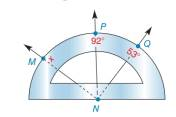Chapter 1.4, Problem 31E### Elementary Geometry for College St...

6th Edition
Daniel C. Alexander + 1 other
ISBN: 9781285195698

#### Solutions

Chapter
Section### Elementary Geometry for College St...

6th Edition
Daniel C. Alexander + 1 other
ISBN: 9781285195698
Textbook Problem
1 views

#Exercises 3 0 , 3 1 On the protractor shown for Exercise 30, ∠ M N P and ∠ P N Q are complementary. Find x .

To determine

To find:

The measure of x.

Explanation

Given:

The given figure is,

MNP and PNQ are complementary.

Definition:

If the sum of two angles is 90° then the angles are known as complementary angles. Each angle in the pair is the complement of the other angle.

If a point say M lies in the interior of PQR, then mPQM+mRQM=mPQR.

Calculation:

Consider the figure (1) with points R and S.

Figure (1)

From figure (1) it can be seen that the measure of SNQ is 53°.

The measure of MNS can be written as,

mMNS=mMNP+mMNQ+mSNQx=mMNP+mMNQ+mSNQ(1)

MNP and PNQ are complementary.

mMNP+mPNQ=90°mMNP=90°mPNQ

### Still sussing out bartleby?

Check out a sample textbook solution.

See a sample solution

#### The Solution to Your Study Problems

Bartleby provides explanations to thousands of textbook problems written by our experts, many with advanced degrees!

Get Started

#### Find the mean for the following set of scores: 2, 7, 9, 4, 5, 3, 0, 6

Essentials of Statistics for The Behavioral Sciences (MindTap Course List)

#### In Problems 7-34, perform the indicated operations and simplify. 23.

Mathematical Applications for the Management, Life, and Social Sciences

#### True or False: y′ + xey = ex+y is separable.

Study Guide for Stewart's Single Variable Calculus: Early Transcendentals, 8th

#### The slope of the tangent line to r = cos θ at is:

Study Guide for Stewart's Multivariable Calculus, 8th